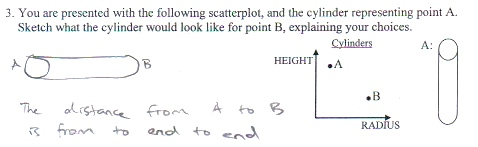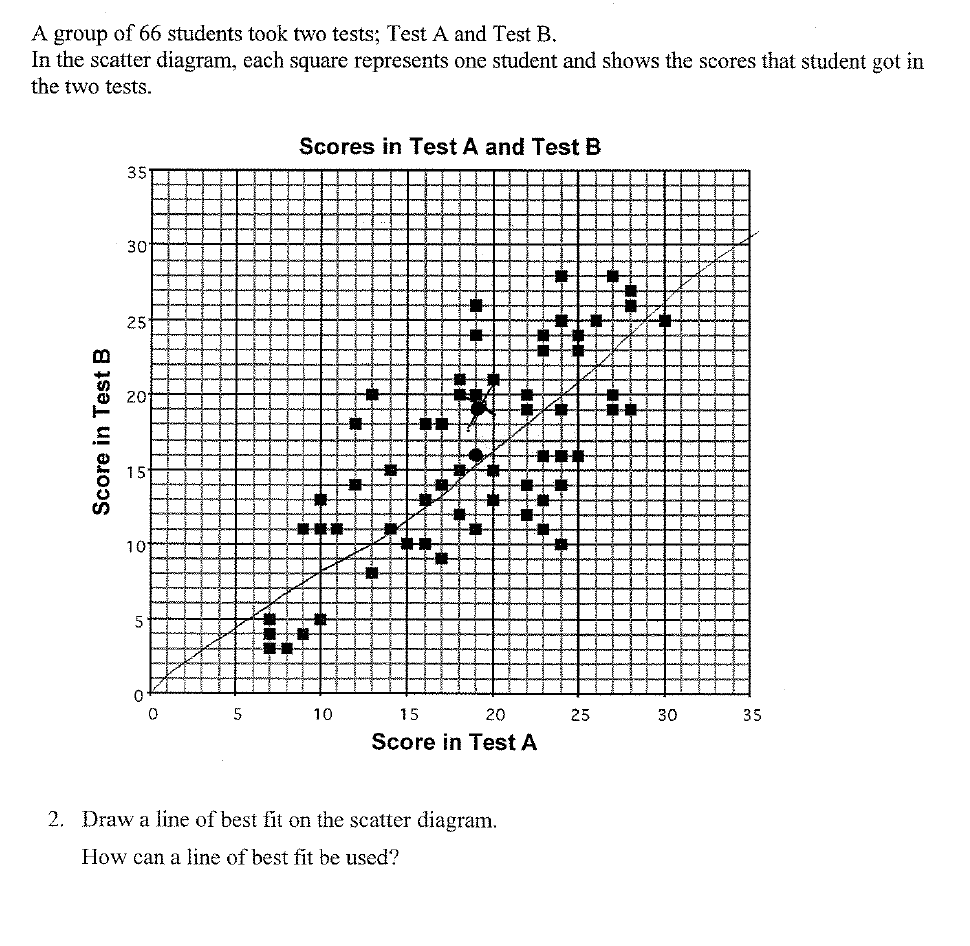Categories

## Cylinder Scatterplot1. This is a really cool question.

2. Gregory Taylor says he doesn’t know what the kid was thinking. Thoughts?

Categories

## Mean (Number of) Girls (and Boys)

“Find the mean number of siblings.”Student 1: (0+1+2+3+4+5)/6 = 2.5
Student 2: (1+4+6+4+2+1)/18=1
Student 3: (1+4+6+4+2+1)/6=3
Student 4: (0+1+2+3+4+5)/18 = 0.83
What sort of misconceptions can you dig out of these student responses? If this were your class, how would you respond?
Categories

## Conceptual Understanding of Line of Best Fit

Here’s a conceptual question (taken from the Shell Centre) that provoked some solid responses from students:Here are a few of the responses: